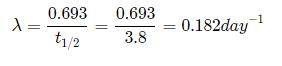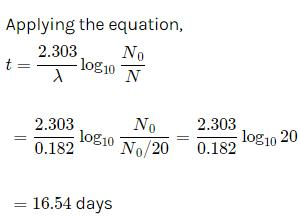Courses

# Test: Nuclei

## 30 Questions MCQ Test Physics Class 12 | Test: Nuclei

Description
This mock test of Test: Nuclei for JEE helps you for every JEE entrance exam. This contains 30 Multiple Choice Questions for JEE Test: Nuclei (mcq) to study with solutions a complete question bank. The solved questions answers in this Test: Nuclei quiz give you a good mix of easy questions and tough questions. JEE students definitely take this Test: Nuclei exercise for a better result in the exam. You can find other Test: Nuclei extra questions, long questions & short questions for JEE on EduRev as well by searching above.
QUESTION: 1

### What percentage of the mass of an atom is concentrated in the nucleus?

Solution:

More than 99.99% of the mass of any atom is concentrated in its nucleus. If the mass of protons and neutrons (which are in the nucleus of every atom) is approximately one atomic mass unit, then the relative mass of an electron is 0.0005 atomic mass units.

QUESTION: 2

### In the mass number range A = 30 to 170, the binding energy per nucleon is

Solution:

The binding energy is a very short order range force.
So beyond a certain amount of range the force has no influence beyond that. that's why after
certain number the binding energy per nucleon do not change even if we add more nucleons.

QUESTION: 3

### Plutonium decays with a half-life of 24000 years. If the plutonium is stored for 72000 years, then the fraction of plutonium that remains is

Solution:

t1/2​=24000 yrs
It is stored for 3 half-lives.
Fraction remaining is (1/2​)3=1/8

QUESTION: 4

In a nuclear reaction which of the following is conserved

Solution:
QUESTION: 5

Cadmium rods are used in a nuclear reactor for

Solution:

In nuclear reactors, fission of uranium is carried out by bombarding  a slow moving neutron on uranium which results in emission of 3 more neutrons along with the byproducts  So, cadmium rods are used to absorb extra neutrons in order to achieve controlled chain reaction.

QUESTION: 6

In what units is mass measured on the atomic scale?

Solution:

The atomic mass of an element is the average mass of the atoms of an element measured in atomic mass unit (amu, also known as daltons, D). The atomic mass is a weighted average of all of the isotopes of that element, in which the mass of each isotope is multiplied by the abundance of that particular isotope.

QUESTION: 7

Energies associated with nuclear processes are

Solution:

Unlike a chemical reaction, a nuclear reaction results in a significant change in mass and an associated change of energy, as described by Einstein’s equation. Nuclear reactions are accompanied by large changes in energy, which result in detectable changes in mass. The change in mass is related to the change in energy according to Einstein’s equation: ΔE = (Δm)c2. Large changes in energy are usually reported in kiloelectronvolts or megaelectronvolts (thousands or millions of electronvolts). With the exception of 1H, the experimentally determined mass of an atom is always less than the sum of the masses of the component particles (protons, neutrons, and electrons) by an amount called the mass defect of the nucleus. The energy corresponding to the mass defect is the nuclear binding energy, the amount of energy released when a nucleus forms from its component particles. In nuclear fission, nuclei split into lighter nuclei with an accompanying release of multiple neutrons and large amounts of energy. The critical mass is the minimum mass required to support a self-sustaining nuclear chain reaction. Nuclear fusion is a process in which two light nuclei combine to produce a heavier nucleus plus a great deal of energy.

QUESTION: 8

B210has a half life of 5 days. The time taken for seven-eighth of a sample to decay is

Solution:

Half-life of Bi210=5 days
∴k= 0.693/(t1/2) ​=(0.693/5) ​day−1
Using k=(2.303/t)​ log (a/a-x)
(where a = a0​, (let) ⇒x=7/8 ​a0​, t is time taken in decay and k is rate constant)
We get, t=(2.303×5/0.693)log a0​/(1/8)a0​​
= (2.303×5/0.693) ​log8=15days

QUESTION: 9

If 10% of a substance decays in 10 days, then approximate percentage of substance left after 24 days is

Solution:

Given, 10% of substance decays in 10 days
So, let [A]o=100, [A]t=100-10=90
So, K=(2.303/t) log ([A]o/[A]t)
K= (2.303/10) log (100/90)
K= (2.303/10) (log10-log9)
K= (2.303/10)91-0.9542)     [log9=0.9542]
K= (2.303/10) x 0.0458 day-1
So, after 24 days,
t= (2.303/K) log (100/[A]t)
24=2.303x10/2.303x0.0458) log [100/[A]t]
log[100/[A]t]=(24x0.0458)/10=0.1099
100/[A]t=antiog(0.1099)=1.288
So, [A]t=100/1.288≈100/1.3
[A]t=76.9≈77%
The closest answer is 78% which is option A. So the correct answer would be option A

QUESTION: 10

Choose the WRONG statement. A thermonuclear fusion reactor is better than a fission reactor for the following reason:

Solution:
QUESTION: 11

A free neutron decays into

Solution:

Neutron is unstable outside the nucleus.
It's decaying equation is given below.
0​n111​H+0−1e+v

QUESTION: 12

The disintegration energy is

Solution:
QUESTION: 13

A radioactive substance has a half life of four months. Three fourths of the substance will decay in

Solution:

Substance left undecayed, N0​−(3/4)​N0​=(1/4)​N0
N/N0​ ​=1/4​=(1/2​)n

∴ Number of atoms left undecided, n=2 i.e, in two half-lives
∴ t=nT=2×4=8 months

QUESTION: 14

At a given time there are 25% undecayed nuclei in a sample. After 10 seconds number of undecayed nuclei reduces to 12.5%. Then mean life of the nuclei will be about

Solution:

Half-life of radioactive sample, i.e., the time in which the number of undecayed nuclei becomes half (T) is 10 s.
Mean life, τ=T/loge​2=10s/0.693=1.443×10=14.43s ≈ 15s

QUESTION: 15

Which of the following particles can be added to the nucleus of an atom without changing its chemical properties?

Solution:

When neutrons are added to the nucleus of an atom its chemical properties remain unchanged because the atomic number of particles remains the same. But when electron, proton or alpha particles are added, atomic number changes so chemical properties change.

QUESTION: 16

Relation between atomic number (Z), neutron number (N) and mass number (A) is

Solution:
QUESTION: 17

α-rays are

Solution:

Alpha particles, also called alpha rays or alpha radiation, consist of two protons and two neutrons bound together into a particle identical to a helium-4 nucleus. They are generally produced in the process of alpha decay, but may also be produced in other ways.

QUESTION: 18

The half life of radon is 3.8 days. After how many days will only one twentieth of a radon sample be left over?

Solution:Let the initial amount of radon be N0​ and the amount left after t days be N which is equal to N0​/2QUESTION: 19

90% of a radioactive sample is left undisintegrated after time τ has elapsed, what percentage of initial sample will decay in a total time 2τ?

Solution:

Given that 90% is left un-decayed after time 't'.
Hence, 10% decays in time 't'.
Initially assume that the amount of substance is 'x'
After time 't' 10% is decayed.
i.e. Amount of substance left =0.9x
After further time 't' another 10% is decayed.
i.e. 0.1×0.9x is decayed
Leaving behind 0.81x.
Hence after time 2t we see that 0.19x has decayed, which is 19%.

QUESTION: 20

The average number of neutrons released by the fission of one uranium atom is

Solution:

Fission also results in the production of several neutrons, typically two or three. On the average, about 2.5 neutrons are released per unit and the energy of a neutron released per fission of a uranium atom are 2 MeV.

QUESTION: 21

The nuclei of isotopes of a given element contain the same number of

Solution:

An isotope is one of two or more forms of the same chemical element. Different isotopes of an element have the same number of protons in the nucleus, giving them the same atomic number, but a different number of neutrons giving each elemental isotope a different atomic weight.

QUESTION: 22

β -rays and γ-rays are respectively

Solution:

Beta radiation ~ Stream of electrons (unit negative charge). Beta positive radiation is when a positron is emitted rather than an electron.
Gamma radiation ~ Electromagnetic radiation of very short wavelength = high photon energy.

QUESTION: 23

Nuclear fusion is possible

Solution:

Nuclear fusion is possible only between light nuclei as they become more stable by acquiring greater atomic number to form particles with intermediate mass. Nuclear fission is possible with heavy nuclei.

QUESTION: 24

What is the main source of energy of the sun?

Solution:

Like most stars, the sun is made up mostly of hydrogen and helium atoms in a plasma state. The sun generates energy from a process called nuclear fusion. During nuclear fusion, the high pressure and temperature in the sun's core cause nuclei to separate from their electrons.

QUESTION: 25

All nuclides with same mass number A are called

Solution:

Nuclides which have the same number of  protons are called isotopes. When nuclides have the same mass, numbers are called isobars. When nuclides have same number of neutron are called isotones. When the elements have the same molecular formula but have different arrangements of atoms in space are called isomers.

QUESTION: 26

When a hydrogen bomb explodes, which of the following is used?

Solution:

The hydrogen bomb is a nuclear weapon that uses a mixture of fission and fusion to produce a massive explosion.
The nuclear fission generates enough heat to initiate the nuclear fusion reaction. After that, the nuclear fusion releases enormous amounts of energy, making the hydrogen bomb a lot more powerful than an atomic bomb.

QUESTION: 27

The half-life T1/2of a radionuclide is

Solution:

The hydrogen bomb is a nuclear weapon that uses a mixture of fission and fusion to produce a massive explosion.
The nuclear fission generates enough heat to initiate the nuclear fusion reaction. After that, the nuclear fusion releases enormous amounts of energy, making the hydrogen bomb a lot more powerful than an atomic bomb.

QUESTION: 28

A radioactive material decays by simultaneous emission of two particles with respective half lives 1620 and 810 years. The time in years, after which one fourth of the material remains is

Solution:

Since, from Rutherford-Soddy law, the number of atoms left after half-lives is given by
N=N0​(1/2​)n
where, N0​ is the original number of atoms.
The number of half-lives, n= time of decay​/effective half−life
Relation between effective disintegration constant (λ) and half-life (T)
λ=ln2/T​
∴λ1​+λ2​= (​ln2/ T1​)+ ​(ln2/ T2​)
Effective half-life,
1/T​=1/T1​​+1/T2​​=(1/1620)​+(1/810)​
1/T​=1+2/1620 ​⇒T=540yr
∴n=T/540
∴N=N0​(1/2​)t/540⇒N/N0​​=(1/2​)2=(1/2​)t/540
⇒t/540​=2⇒t=2×540=1080yr

QUESTION: 29

The chemical behavior of an atom depends upon

Solution:

The chemical behavior of atoms is determined by the number and the configuration of the electrons in the atom. The number of protons in the nucleus determines the number of electrons in the atom.

QUESTION: 30

Nuclear mass M is found to be

Solution:

The actual mass is always less than the sum of the individual masses of the constituent protons and neutrons because energy is removed when the nucleus is formed. This energy has mass, which is removed from the total mass of the original particles.